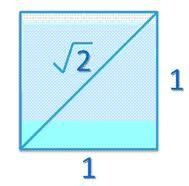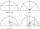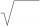# Number

Is number 5.146852 irrational?

Result

#### Solution:

$5.14\stackrel{‾}{6852}=\frac{514}{100}+\frac{6852}{1000000-100}=\frac{389941}{75763}\in Q$We would be pleased if you find an error in the word problem, spelling mistakes, or inaccuracies and send it to us. Thank you!## Next similar math problems:

• Numeric questionIt is possible negative irrational number?
• Transforming cuboidCuboid with dimensions 6 cm, 10, and 11 cm is converted into a cube with the same volume. What is its edge length?
• LogsTrunk diameter is 52 cm. Is it possible to inscribe a square prism with side 36 cm?
• Expression 6Evaluate expression: -6-2(4-8)-9
• RootsCalculate the square root of these numbers: